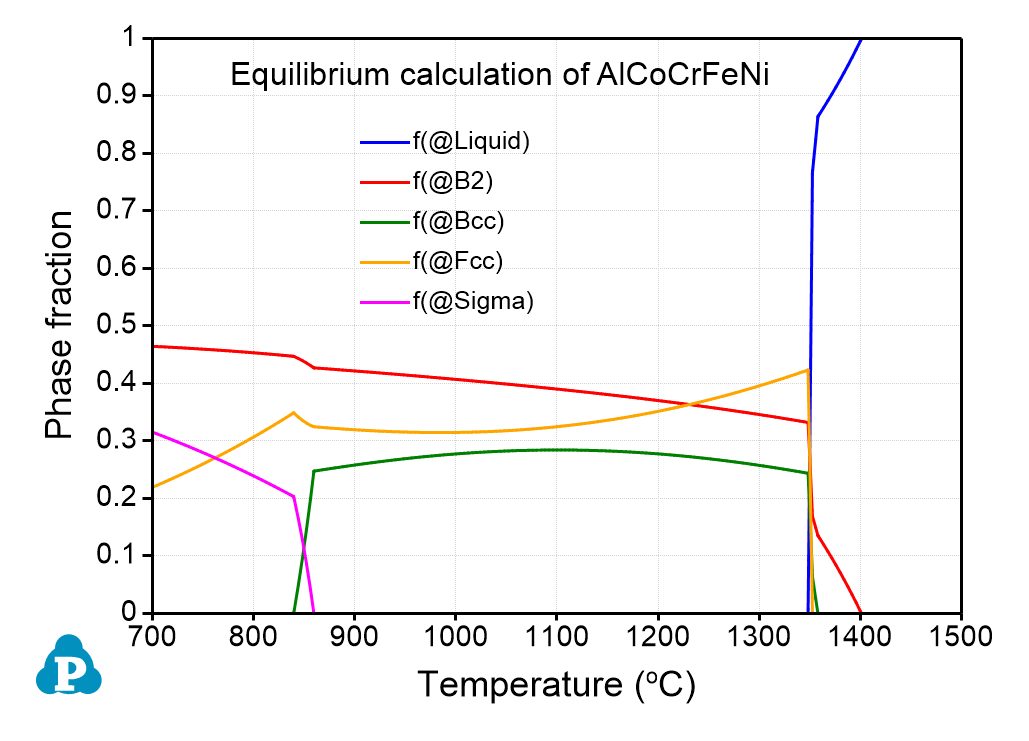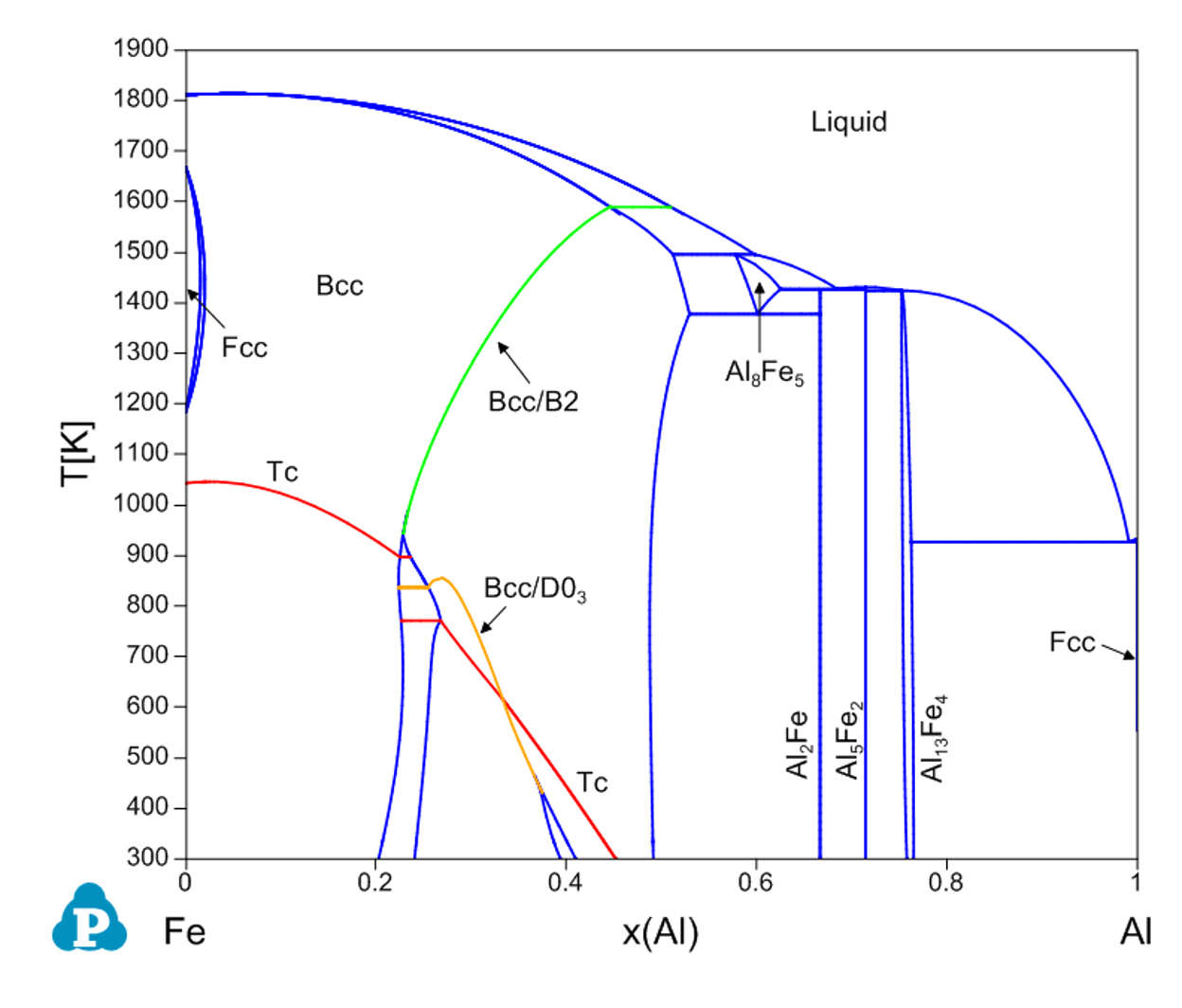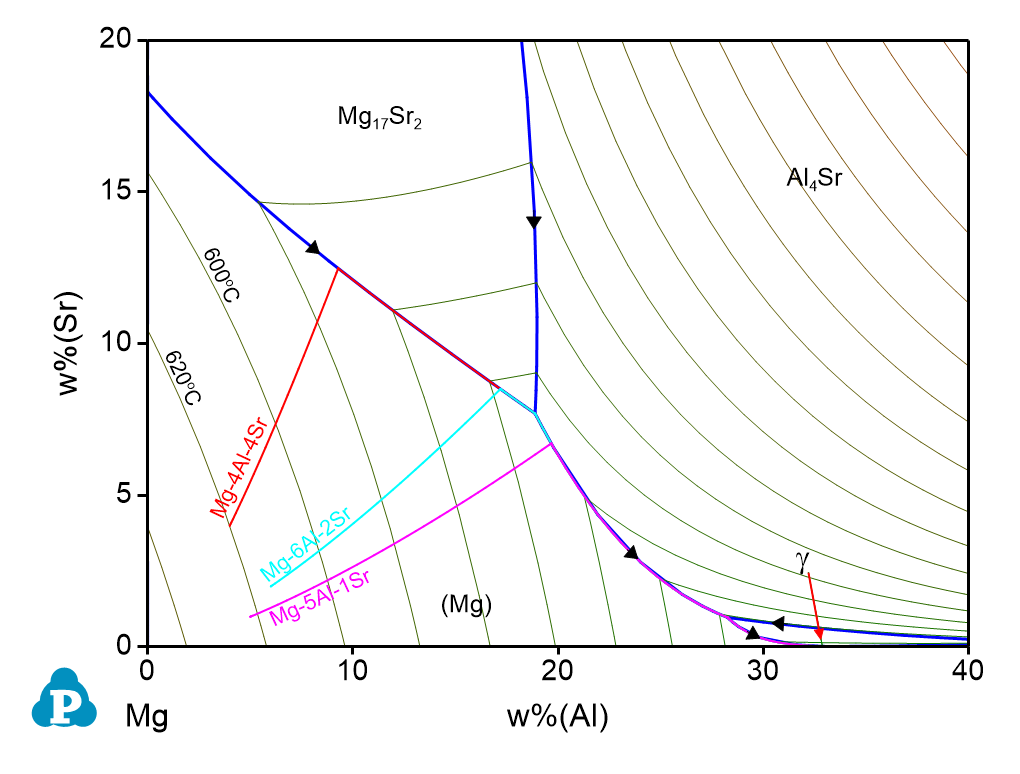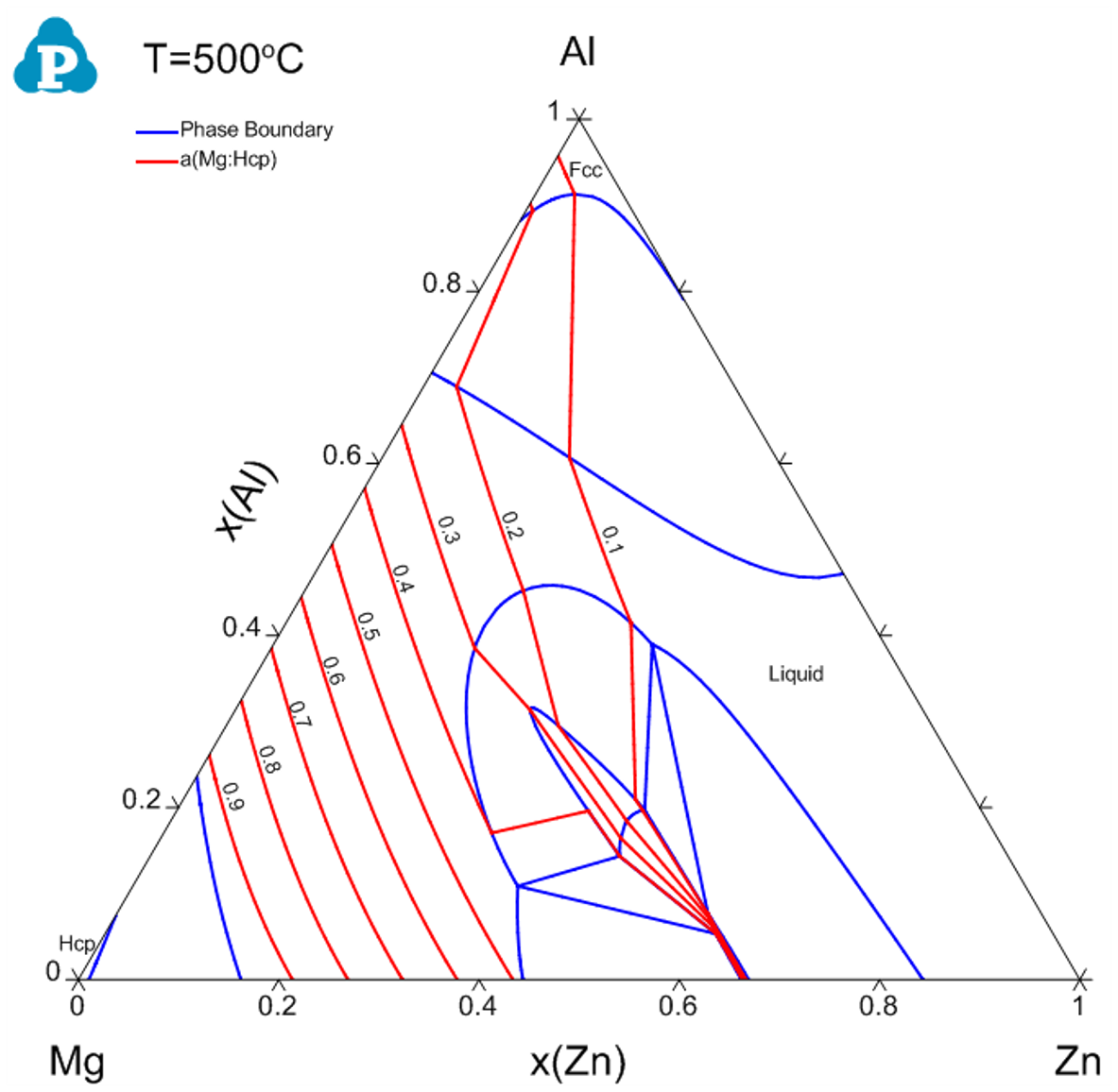## PanPhaseDiagram

PanPhaseDiagram: Phase diagram and thermodynamic calculations view documentations

PanPhaseDiagram module is for the calculation of phase equilibria and thermodynamic properties of multi-component, multi-phase systems. In addition to the stable and metastable phase equilibria, phase transformation temperature, phase fraction, and thermodynamic properties, such as Gibbs energy, enthalpy, entropy and chemical driving force, can all be readily calculated. This module can also be used for solidification simulation based on Scheil model and Lever rule, and provide latent heat and total heat evolved during solidification. A thermodynamic database for the system is required for the PanPhaseDiagram module to obtain the sensible phase equilibria and thermodynamic properties of the alloy system of interest. Additional mobility database is needed to calculate mobility and diffusivity, and additional property database (such as molar volume database) is needed to calculate the respective properties. Please refer to Databases for more information.

Basic Functionalities of PanPhaseDiagram Module

• Phase diagrams: isotherm, isopleth, phase projection for an n-component system, stability diagram, 3D phase diagram, pseudo-binary section, pressure diagram
• Property contour diagrams: contour diagrams for thermodynamic properties and physical properties, such as activity, phase fraction, density, driving force and surface tension
• Phase equilibria: stable and metastable phase equilibria
• Phase properties: amounts and compositions of phases, phase transformation temperatures
• Solidification: solidification path and heat evolution using the Scheil and Lever-rule models
• Thermochemical properties: enthalpy of formation, activity, partial pressure, partial molar properties, excess properties and driving force
• Thermophysical properties: molar volume, density, surface tension and viscosity
• Special properties: magnetic transition, second order phase transformation, zero-phase-fraction lines, reaction equations, spinodal decomposition curve, electrical and thermal conductivity
• Mobility and diffusivities: self, tracer, chemical and inter-diffusivities

Featured Plots of PanPhaseDiagram ModuleThis figure shows an equilibrium calculation of the AlCoCrFeNi high entropy alloy in the temperature range from 700 to 1500oC. The plot shows the fraction of each phase as a function of temperature. The calculated melting temperature of this alloy is about 1400°C. The Fcc, Bcc and B2 phases coexist in the temperature range between 840°C and 1340°C. The fraction of the B2 phase increases with decreasing temperature, while that of the Fcc phase shows the opposite trend. The σ phase starts to form at 880°C and its amount increases at the expense of disordered Bcc and Fcc phases. The Bcc phase disappears at ~840 °C.This figure shows the calculated Fe-Al binary phase diagram. The red dash line is the curie temperature Tc. The green line is the second order transition curve between Bcc and B2, while the yellow line is the second order transition curve between B2 and D03.This figure shows the liquidus projection of the Mg-Al-Sr ternary system at the Mg-corner. The solidification path plotted in the figure can be used to predict the microstructure of cast magnesium alloy for the selected alloy composition.This figure is a contour diagram of Mg activity in the Al-Mg-Zn ternary system at 500oC.